UPSC  >  Practice Test: Pie Chart - 2

# Practice Test: Pie Chart - 2 - UPSC

Test Description

## 10 Questions MCQ Test CSAT Preparation - Practice Test: Pie Chart - 2

Practice Test: Pie Chart - 2 for UPSC 2023 is part of CSAT Preparation preparation. The Practice Test: Pie Chart - 2 questions and answers have been prepared according to the UPSC exam syllabus.The Practice Test: Pie Chart - 2 MCQs are made for UPSC 2023 Exam. Find important definitions, questions, notes, meanings, examples, exercises, MCQs and online tests for Practice Test: Pie Chart - 2 below.
Solutions of Practice Test: Pie Chart - 2 questions in English are available as part of our CSAT Preparation for UPSC & Practice Test: Pie Chart - 2 solutions in Hindi for CSAT Preparation course. Download more important topics, notes, lectures and mock test series for UPSC Exam by signing up for free. Attempt Practice Test: Pie Chart - 2 | 10 questions in 10 minutes | Mock test for UPSC preparation | Free important questions MCQ to study CSAT Preparation for UPSC Exam | Download free PDF with solutions
 1 Crore+ students have signed up on EduRev. Have you?
Practice Test: Pie Chart - 2 - Question 1

### Instructions  Chart 1 shows the distribution by value of top 6 suppliers of MFA Textiles in 1995. Chart 2 shows the distribution by quantity of top 6 suppliers of MFA Textiles in 1995. The total value is 5760 million Euro (European currency). The total quantity is 1.055 million tonnes.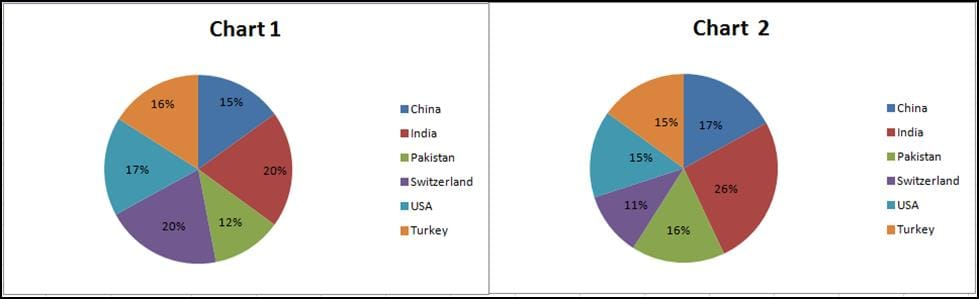Q. The country which has the highest average price is

Detailed Solution for Practice Test: Pie Chart - 2 - Question 1

The country which has the highest average price would have low quality and high value when compared to other countries..

Switzerland fits the above description.

Hence, option A is the answer.

Practice Test: Pie Chart - 2 - Question 2

### Instructions  Chart 1 shows the distribution by value of top 6 suppliers of MFA Textiles in 1995. Chart 2 shows the distribution by quantity of top 6 suppliers of MFA Textiles in 1995. The total value is 5760 million Euro (European currency). The total quantity is 1.055 million tonnes.Q. The average price in Euro per kilogram for Turkey is roughly

Detailed Solution for Practice Test: Pie Chart - 2 - Question 2

Turkey has a value of 16% and a quantity of 15%.

The average price in Euro per kilogram for Turkey is  (16*5.760)/(15*1.055) which is nearly equal to 5.6.

Hence option D.

Practice Test: Pie Chart - 2 - Question 3

### Instructions: For the following three questions: Answer the questions based on the pie charts given below. Chart 1 shows the distribution of 12 million tonnes of crude oil transported through different modes over a specific period of time. Chart 2 shows the distribution of the cost of transporting this crude oil. The total cost was Rs. 30 million.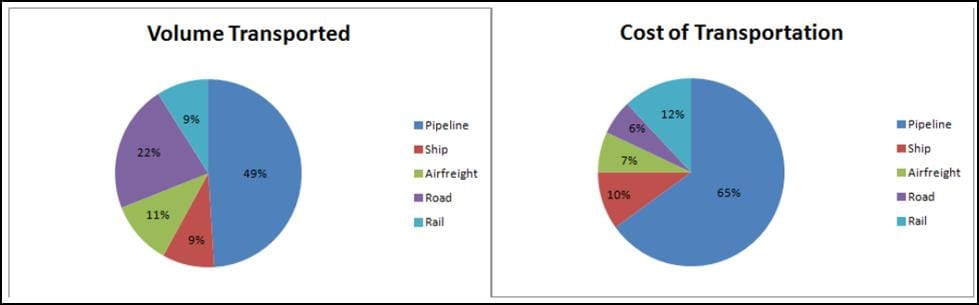Q. The cost in rupees per tonne of oil moved by rail and road happens to be roughly

Detailed Solution for Practice Test: Pie Chart - 2 - Question 3

Total tonnes of transportation by both rail and road is about  31*12/100 = 3.72 million tonnes and total cost incured 18*30/100 = 5.4 .

Hence required value is 5.4/3.72 which is about 1.5.

Hence option B .

Practice Test: Pie Chart - 2 - Question 4

Instructions:
For the following three questions: Answer the questions based on the pie charts given below. Chart 1 shows the distribution of 12 million tonnes of crude oil transported through different modes over a specific period of time. Chart 2 shows the distribution of the cost of transporting this crude oil. The total cost was Rs. 30 million.Q. From the charts given, it appears that the cheapest mode of transport is

Detailed Solution for Practice Test: Pie Chart - 2 - Question 4

Cheapest mode of transport will be the one which will have highest transport volume and comparatively lowest cost.

We can figure out from the graph that Road have wide gap with very less costs.

For Rail, Cost = 12/9

For Pipeline, = 65/49

For Ship, Cost = 10/9

Practice Test: Pie Chart - 2 - Question 5

Instructions:
For the following three questions: Answer the questions based on the pie charts given below. Chart 1 shows the distribution of 12 million tonnes of crude oil transported through different modes over a specific period of time. Chart 2 shows the distribution of the cost of transporting this crude oil. The total cost was Rs. 30 million.Q. If the costs per tonne of transport by ship, air and road are represented by P, Q and R respectively, which of the following is true?

Detailed Solution for Practice Test: Pie Chart - 2 - Question 5

If the costs per tonne of transport by ship, air and road are represented by P, Q and R respectively.

P = 10/9
Q = 7/11
R = 6/22

We can see that P>Q and Q>R  and P>R.

Practice Test: Pie Chart - 2 - Question 6

Total revenues are Rs. 1800 crores. Overall profit margin is 10%. The division with the largest revenue has the least profit margin but not the least profits. The division with the profit margin higher than all others generates the least profit. Exactly one division has the same profit margin as the overall Company. Company D generates more profits than Company E.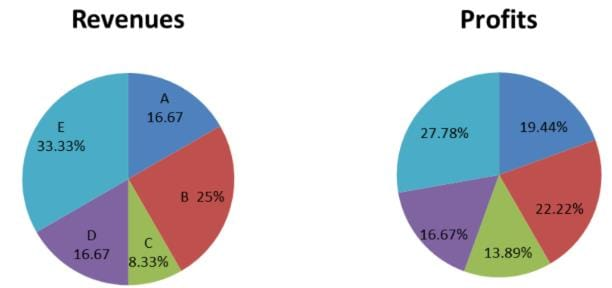Q. How much profit did Company A make?

Practice Test: Pie Chart - 2 - Question 7

Total revenues are Rs. 1800 crores. Overall profit margin is 10%. The division with the largest revenue has the least profit margin but not the least profits. The division with the profit margin higher than all others generates the least profit. Exactly one division has the same profit margin as the overall Company. Company D generates more profits than Company E.Q. What was the profit margin for company B?

Practice Test: Pie Chart - 2 - Question 8

Total revenues are Rs. 1800 crores. Overall profit margin is 10%. The division with the largest revenue has the least profit margin but not the least profits. The division with the profit margin higher than all others generates the least profit. Exactly one division has the same profit margin as the overall Company. Company D generates more profits than Company E.Q. How much profit did company E make?

Practice Test: Pie Chart - 2 - Question 9

The following pie-chart shows the percentage distribution of the expenditure incurred in publishing a book. Study the pie-chart and the answer the questions based on it.

Various Expenditures (in percentage) Incurred in Publishing a Book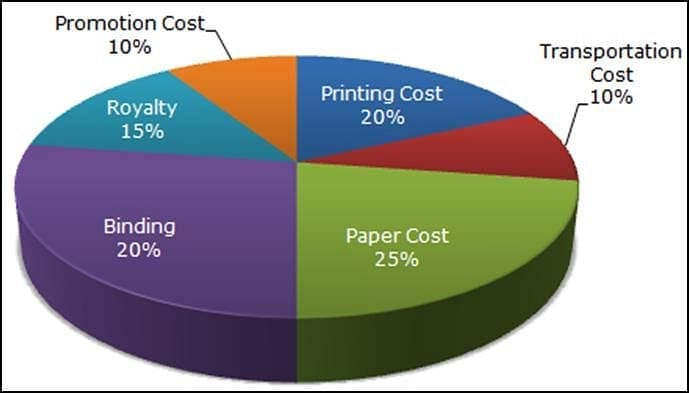Q. If for a certain quantity of books, the publisher has to pay Rs. 30,600 as printing cost, then what will be amount of royalty to be paid for these books?

Detailed Solution for Practice Test: Pie Chart - 2 - Question 9

Let the amount of Royalty to be paid for these books be Rs. r.

Then, 20 : 15 = 30600 : r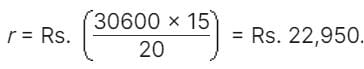Practice Test: Pie Chart - 2 - Question 10

The following pie-chart shows the percentage distribution of the expenditure incurred in publishing a book. Study the pie-chart and the answer the questions based on it.

Various Expenditures (in percentage) Incurred in Publishing a BookQ. The price of the book is marked 20% above the C.P. If the marked price of the book is Rs. 180, then what is the cost of the paper used in a single copy of the book?

Detailed Solution for Practice Test: Pie Chart - 2 - Question 10

Clearly, marked price of the book = 120% of C.P.

Also, cost of paper = 25% of C.P

Let the cost of paper for a single book be Rs. n.

Then, 120 : 25 = 180 : n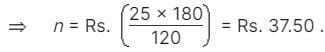## CSAT Preparation

197 videos|151 docs|200 tests
Information about Practice Test: Pie Chart - 2 Page
In this test you can find the Exam questions for Practice Test: Pie Chart - 2 solved & explained in the simplest way possible. Besides giving Questions and answers for Practice Test: Pie Chart - 2, EduRev gives you an ample number of Online tests for practice

## CSAT Preparation

197 videos|151 docs|200 tests

### How to Prepare for UPSC

Read our guide to prepare for UPSC which is created by Toppers & the best Teachers